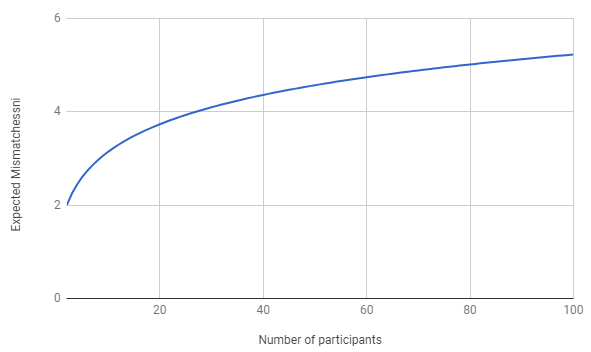Each of seven dudes sleeps in his own bed in a shared dormitory. Every night, they retire to bed one at a time, always in the same sequential order, with the youngest dude retiring first and the oldest retiring last. On a particular evening, the youngest dude is in a jolly mood. He decides not to go to his own bed but rather to choose one at random from among the other six beds. As each of the other dudes retires, he chooses his own bed if it is not occupied, and otherwise chooses another unoccupied bed at random.

1. What is the probability that the oldest dude sleeps in his own bed?
2. What is the expected number of dudes who do not sleep in their own beds?

(fivethirtyeight; I’ve switched to dudes from “dwarfs,” in recognition that the latter term is not cared for by many people who are differently tall.)

### Solution

The stipulation that the young dude chooses among the other beds introduces a complication that slightly muddies what is otherwise a very pretty problem. Call the “pretty version” of the problem the variation in which he can also end up in his own bed.

Let’s start with the pretty version of the problem. We’ll work with $n$ dudes and beds, and label the dudes and beds $1$ through $n$. When dude $k$ arrives ($k\geq 2$), all $k-2$ beds from $2$ to $k-1$ are occupied (if dudes $2$ to $k-1$ had found them unoccupied, they’d have chosen them). One more bed is occupied among the other $n-k+2$ beds (which are beds $1,k,k+1,\ldots,n$) and it is occupied by a dude who chose it randomly. Bed $k$ is as likely as any of those to be the occupied one, and so the chance that it is occupied, which is also the chance that dude $k$ ends up mismatched, is:

$P^{pretty}_k = \frac{1}{n-k+2}$

So the mismatch probabilities for dudes $2,\ldots,n$ are $\frac{1}{n},\frac{1}{n-1},\ldots,\frac{1}{3},\frac{1}{2}$. (The probability that dude $1$ is mismatched is $\frac{n-1}{n}$.)

Asking for the probability that dude $k$ is mismatched in the puzzle as actually posed is equivalent to asking, in the pretty version, for the probability that dude $k$ is mismatched given that at least one dude is mismatched. We find this as follows (where $M$ means that there is at least one mismatch, $K$ means that dude $k$ is mismatched, and $P(A|B)$ is the conditional probability of $A$ given $B$):

$P^{pretty}_k = P(M)P(K|M) + P(\neg M)P(K | \neg M)$ $= \frac{n-1}{n}P^{as-posed}_k + \frac{1}{n}\cdot 0 = \frac{1}{n-k+2}$ $P^{as-posed}_k = \frac{n}{(n-1)}\cdot\frac{1}{n-k+2}$

For $7$ dudes and beds, then, the oldest dude has probability $5/12$ of sleeping in his own bed.

Now that we know, for both the simple and as-posed versions of the puzzle, how likely each dude is to be mismatched, we can compute the expected number of mismatched dudes by simply adding the probabilities for all $n$ dudes together. This works because the probability of a mismatch in a given bed is also the expected number of mismatches in that bed, and the expectation of any sum of values (in this case the sum of the numbers of mismatches in each of the beds) is the sum of the expectations of the individual values.

$E^{pretty}_n = \sum_{k=1}^n P^{pretty}_k = \frac{n-1}{n}+\sum_{k=2}^n \frac{1}{n-k+2} = \sum_{i=1}^{n-1} \frac{1}{i} = H_{n-1}$

Here $H_n$ is the $n$th harmonic number (the sum of the reciprocals of the first $n$ counting numbers). The harmonic numbers increase more and more slowly, but are unbounded, so there is no limit to the expected mismatches for large $n$.

To get the expectation for the problem as posed, we simply need to multiply by the constant factor $\frac{n}{n-1}$ to account for the difference in every dude’s (including dude $1$’s!) mismatch probability in the sum, yielding:

$E^{as-posed}_n = \frac{n}{n-1}H_{n-1}$

Here are some values for small $n$ (the exact expectation for seven dudes, by the way, is $343/120$ in the problem as posed, or $49/20$ in the pretty version):

n $E^{as-posed}_n$
2 2.0
3 2.25
4 2.44444444444
5 2.60416666667
6 2.74
7 2.85833333333
8 2.96326530612
9 3.05758928571
10 3.14329805996

The values are confirmed by this Monte Carlo simulation (in Python).

from random import randint
Reps = 10000000
for N in range(3,11):
Accum = 0
for _ in range(Reps):
OpenBeds = list(range(N))
MatchCount = 0
del OpenBeds[randint(1,N-1)]
for i in range(1,N):
if i in OpenBeds:
OpenBeds.remove(i)
MatchCount += 1
else:
del OpenBeds[randint(0,N-i-1)]
Accum += N-MatchCount
print N, ",", 1.0*Accum/Reps